\u200E
NLP预训练模型小型化与部署的那些事儿6月，百度飞桨 & 自然语言处理部携手推出了12节NLP视频课，课程中详细讲解了本实践项目。

https://aistudio.baidu.com/aistudio/course/introduce/24177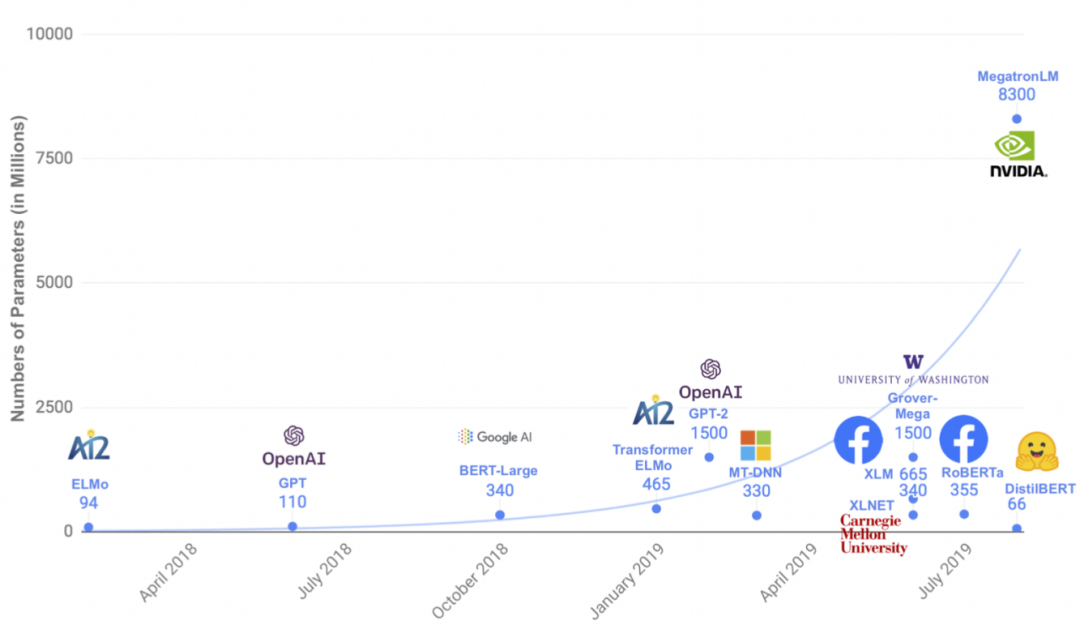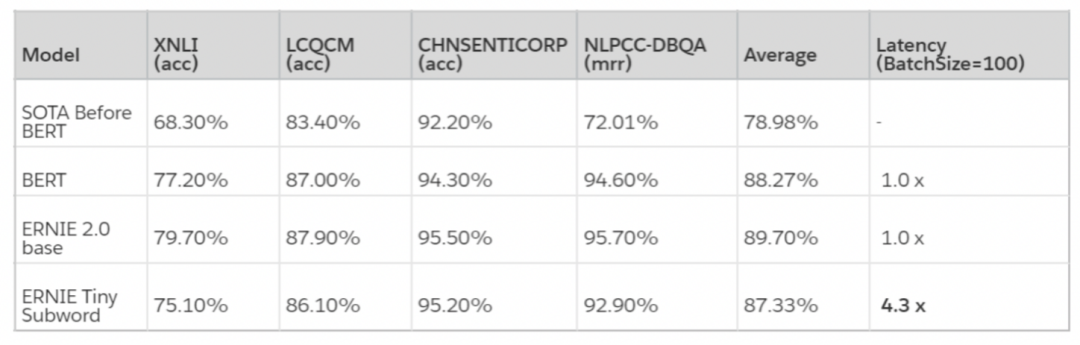ERNIE-Tiny 微调

ERNIE-Tiny是使用ERNIE 2.0 Base模型经通用蒸馏得到的轻量级模型，蒸馏时使用蒸馏信号（知识）包括ERNIE教师模型的预测结果（logits或软标签，这能够表示更细粒度的类别概率信息，提供信息量更大的知识）和中间各层的结果（包括中间层的输出表征和attention分布）。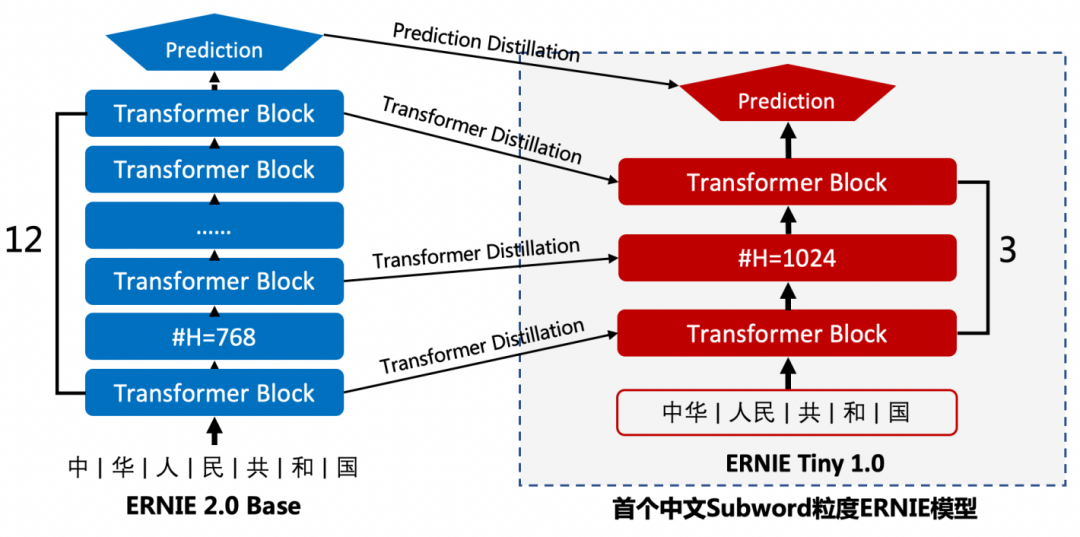ERNIE-Tiny更浅（12层->3层transformer block）、更短（字粒度->subword粒度缩短输入长度），并加大宽度（768->1024 hidden size）弥补模型变浅带来的效果损失，在4倍提速的同时模型效果只有少量下降。直接使用ERNIE-Tiny在下游任务上微调即可得到兼顾效果与性能的模型。

``from paddlenlp.transformers import ErnieTinyTokenizer, ErnieForSequenceClassification# 创建Ernie Tiny模型使用的tokenziertokenizer = ErnieTinyTokenizer.from_pretrained('ernie-tiny')# 创建使用Ernie Tiny预训练模型的句子分类模型model = ErnieForSequenceClassification.from_pretrained('ernie-tiny', num_classes=2)# 打印查看Ernie Tiny``

ERNIE 蒸馏 Bi-LSTM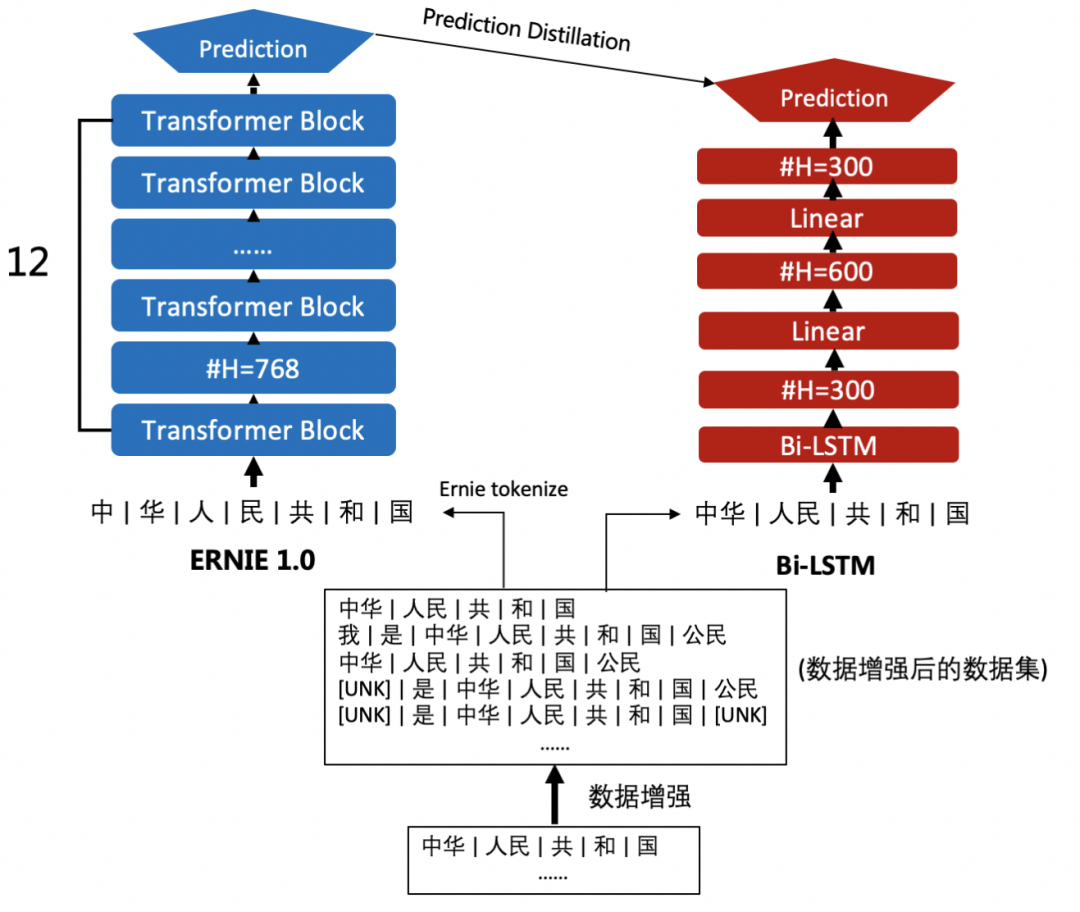2.1 数据准备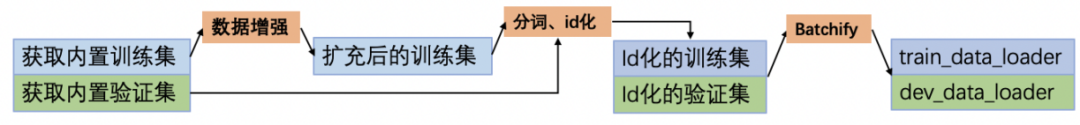``from paddlenlp.datasets import load_dataset# 获取内置的数据集train_ds, dev_ds = load_dataset('chnsenticorp', splits=["train", "dev"])``

• n-gram sampling，即以一定的概率从句子中采样n-gram作为样本，其中n的范围可进行设置。（类似图像领域的随机裁剪）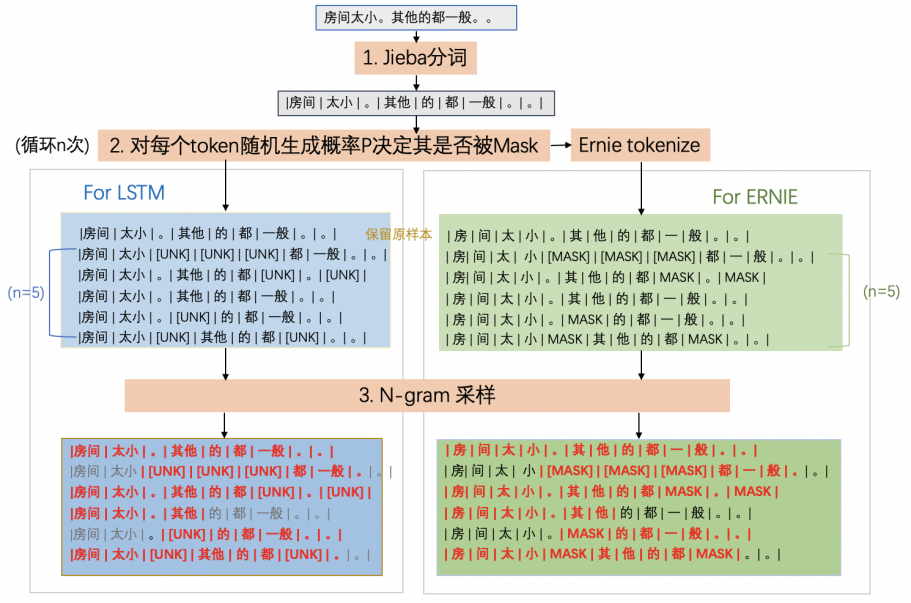``# 教师模型Ernie使用的tokenizertokenizer = ErnieTokenizer.from_pretrained("ernie-1.0")def apply_data_augmentation(data,                            n_iter=2,                            p_mask=0.1,                            p_ng=0.25,                            ngram_range=(2, 10)):    # 固定seed便于演示复现    np.random.seed(2021)    # 存放扩充后的数据    new_data = []    # 循环对数据集的每条样本进行数据增强    for example in data:        # 使用jieba分词，基于词粒度进行数据增强        words = [word for word in jieba.cut(example['text'])]        # 学生模型使用jieba分词结果，教师模型使用ErnieTokenizer结果        lstm_tokens, ernie_tokens = words, tokenizer.tokenize(example['text'])        # 将原样本数据加入扩充数据集        new_data.append({            "lstm_tokens": lstm_tokens,            "ernie_tokens": ernie_tokens,            "label": example['label']        })        for _ in range(n_iter):            # 存放数据增强后学生模型和教师模型的tokenize结果            lstm_tokens, ernie_tokens = [], []            # 1. Masking            for word in words:                # 基于词粒度，词内容整体list处理                if np.random.rand() < p_mask:                    lstm_tokens.append(['[UNK]'])                    ernie_tokens.append([tokenizer.mask_token])                else:                    lstm_tokens.append([word])                    ernie_tokens.append(tokenizer.tokenize(word))            # 2. N-gram sampling            if np.random.rand() < p_ng:                ngram_len = min(np.random.randint(ngram_range, ngram_range + 1), len(words))                start = np.random.randint(0, len(words) - ngram_len + 1)                lstm_tokens = lstm_tokens[start:start + ngram_len]                ernie_tokens = ernie_tokens[start:start + ngram_len]            # 展开得到tokenize的结果：            # lstm:[[房间], [太小]] -> [房间, 太小] ernie:[[房, 间], [太, 小]] -> [房, 间, 太, 小]            lstm_tokens, ernie_tokens = list(chain(*lstm_tokens)), list(chain(*ernie_tokens))            # 将新样本加入扩充数据集            new_data.append({                "lstm_tokens": lstm_tokens,                "ernie_tokens": ernie_tokens,                "label": example['label']            })    return new_data``

``# 执行数据增强，`batched=True`时执行`data_aug_fn(train_ds)`train_ds = train_ds.map(apply_data_augmentation, batched=True)``

tokenize 与 id 化

``# 学生模型id转换使用的词典vocab = Vocab.load_vocabulary(    filepath='senta_word_dict_subset.txt',    unk_token='[UNK]',    pad_token='[PAD]')def convert_example_for_lstm(example, max_seq_length=128, is_tokenized=True):    """    将单条样本转成学生模型（Bi-LSTM）需要的输入    """    # tokenize分词    words = example['lstm_tokens'] if is_tokenized else list(jieba.cut(example['text']))    # 使用vocab转为id数据    input_ids = [vocab[word] for word in words][:max_seq_length]    valid_length = np.array(len(input_ids), dtype='int64')    return input_ids, valid_length, np.array(example['label'], dtype="int64")def convert_example_for_ernie(example, max_seq_length=128):    """    将单条样本转成教师模型（ERNIE）需要的输入    """    example = tokenizer(example['ernie_tokens'], max_seq_len=max_seq_length, is_split_into_words=True)    return example['input_ids'], example['token_type_ids']def convert_example_for_distill(example):    """    将单条样本同时转成大小模型均需要的输入    """    # 得到Ernie的输入    ernie_inputs = convert_example_for_ernie(example)    # 得到Bi-LSTM的输入    lstm_inputs = convert_example_for_lstm(example)    return ernie_inputs + lstm_inputs``

``from functools import partial# 训练集转换，`lazy=True`时在实际取数据时进行转换train_ds = train_ds.map(convert_example_for_distill, lazy=True)# 验证集转换dev_ds = dev_ds.map(partial(convert_example_for_lstm, is_tokenized=False), lazy=True)``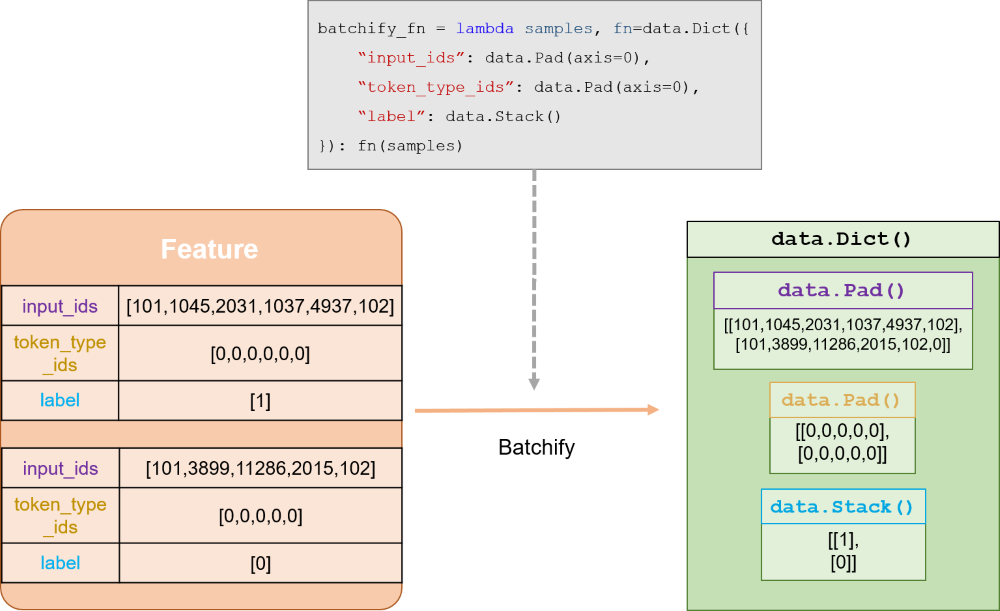2.2 模型定义

ERNIE 教师模型

``from paddlenlp.transformers import ErnieForSequenceClassification# 导入已微调训练好的教师模型teacher = ErnieForSequenceClassification.from_pretrained("/home/aistudio/data/data88390/")``

Bi-LSTM 学生模型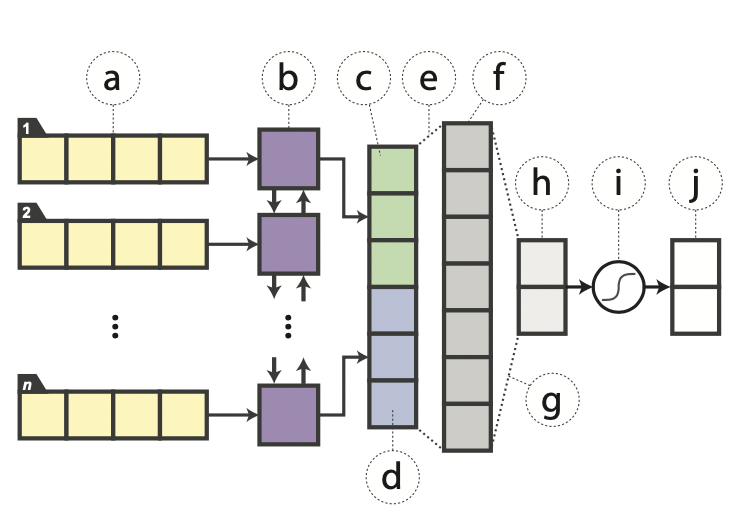``class BiLSTM(nn.Layer):    def __init__(self,vocab_size,embed_dim,hidden_size,output_dim,num_layers=1,                 dropout_prob=0.0,                 padding_idx=0,                 init_scale=0.1):        super(BiLSTM, self).__init__()        self.embedder = nn.Embedding(vocab_size, embed_dim, padding_idx)        self.lstm = nn.LSTM(            embed_dim,            hidden_size,            num_layers,            'bidirect', # 双向LSTM            dropout=dropout_prob)        self.fc = nn.Linear(            hidden_size * 2,            hidden_size,            weight_attr=paddle.ParamAttr(initializer=I.Uniform(                low=-init_scale, high=init_scale)))        self.output_layer = nn.Linear(            hidden_size,            output_dim,            weight_attr=paddle.ParamAttr(initializer=I.Uniform(                low=-init_scale, high=init_scale)))    def forward(self, x, seq_len):        # 对文本输入接入Embedding层        x_embed = self.embedder(x)        # 文本表示、文本长度作为双向LSTM的输入，经过计算得到LSTM的输出和终态表示        lstm_out, (hidden, _) = self.lstm(            x_embed, sequence_length=seq_len)        # 将终态两个方向最后一层的隐状态拼接在一起        out = paddle.concat((hidden[-2, :, :], hidden[-1, :, :]), axis=1)        # 经过一层线性层和Tanh激活函数        out = paddle.tanh(self.fc(out))        # 经过最后一层线性层得到logit并返回        logits = self.output_layer(out)        return logits# 根据超参创建Bi-LSTM学生模型student = BiLSTM(vocab_size, emb_dim, hidden_size,            output_dim, num_layers, dropout_prob,            padding_idx)``

2.3 优化策略与模型训练

``# 定义损失函数mse_loss = nn.MSELoss()# 定义优化器optimizer = paddle.optimizer.Adadelta(    learning_rate=1.0, rho=0.95, parameters=student.parameters())# 教师模型设置为eval模式teacher.eval()# 定义评估指标与评估函数metric = paddle.metric.Accuracy()best_acc = 0def evaluate(model, metric, data_loader):    model.eval()    metric.reset()    for i, batch in enumerate(data_loader):        input_ids, seq_len, labels = batch        logits = model(input_ids, seq_len)        # 每个step调用compute和update        correct = metric.compute(logits, labels)        metric.update(correct)    # 最后调用accumulate得到最后的评价指标（ACC）值    res = metric.accumulate()    model.train()    return resmax_step = 2for epoch in range(epochs):    for i, batch in enumerate(train_data_loader):        global_step += 1        # 从data loader中取出一个batch data        teacher_input_ids, teacher_segment_ids, student_input_ids, seq_len, labels = batch        # 教师模型执行前向计算，获取logits作为蒸馏信号。`no_grad`下的内容将不进行梯度计算        with paddle.no_grad():            teacher_logits = teacher(teacher_input_ids, teacher_segment_ids)        # 学生模型执行前向计算        logits = student(student_input_ids, seq_len)        # 计算学生模型和教师模型logits输出的均方误差损失        loss = mse_loss(logits, teacher_logits)        # 学生模型执行反向计算获取梯度，梯度计算及参数更新、优化器更新        loss.backward()        # 优化器更新学生模型的参数权重，然后清空梯度        optimizer.step()        optimizer.clear_grad()``

Accuracy(%)

ERNIE-1.0

95.55

Bi-LSTM

92.00

Distilled Bi-LSTM

93.333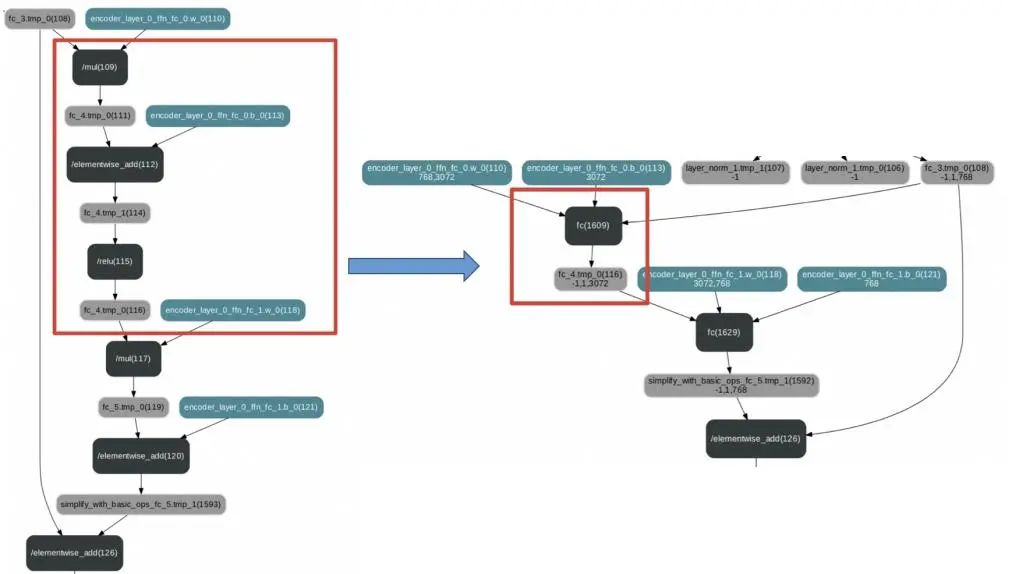3.1 动转静导出模型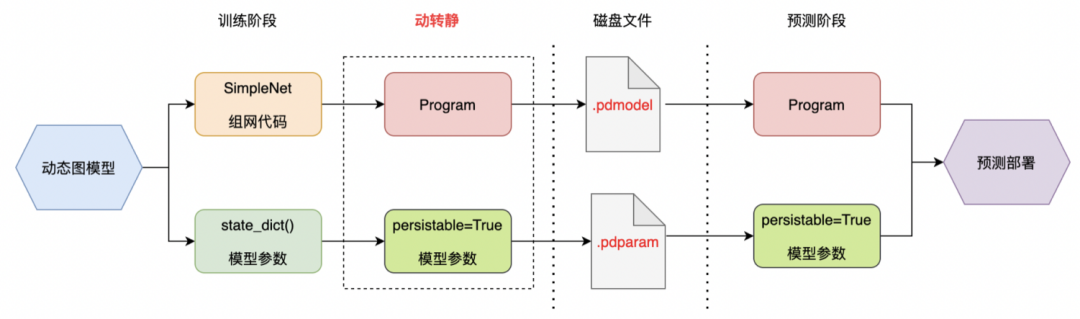``import paddle # 默认为动态图模式，这里开启静态图模式paddle.enable_static() # 定义输入变量，静态图下变量只是一个符号化表示，并不像动态图 Tensor 那样持有实际数据x = paddle.static.data(shape=[None, 128], dtype='float32', name='x')linear = paddle.nn.Linear(128, 256, bias_attr=False)# 定义计算网络，输入和输出也都是符号化表示y = linear(x)# 打印 programprint(paddle.static.default_main_program())# 关闭静态图模式paddle.disable_static()``

• 参数权重：将动态图模型的参数在生成Program时对应到其中的变量上。

``# 设置log输出转写的代码内容# paddle.jit.set_code_level(100)# 加载动态图模型param_state_dict = paddle.load("best_model_7380_933.pdparams")student.set_state_dict(param_state_dict)# 动转静，通过`input_spec`给出模型所需输入数据的描述，shape中的None代表可变的大小，类似上面静态图模式中的`paddle.static.data`model = paddle.jit.to_static(    student,    input_spec=[        paddle.static.InputSpec(            shape=[None, None], dtype="int64"),  # input_ids: [batch_size, max_seq_len]        paddle.static.InputSpec(            shape=[None], dtype="int64")  # length: [batch_size]    ])``

• 网络结构：以.pdmodel为扩展名的文件，可以使用visualdl来可视化。
• 参数权重：以.pdiparams为扩展名的文件。

3.2 使用推理库预测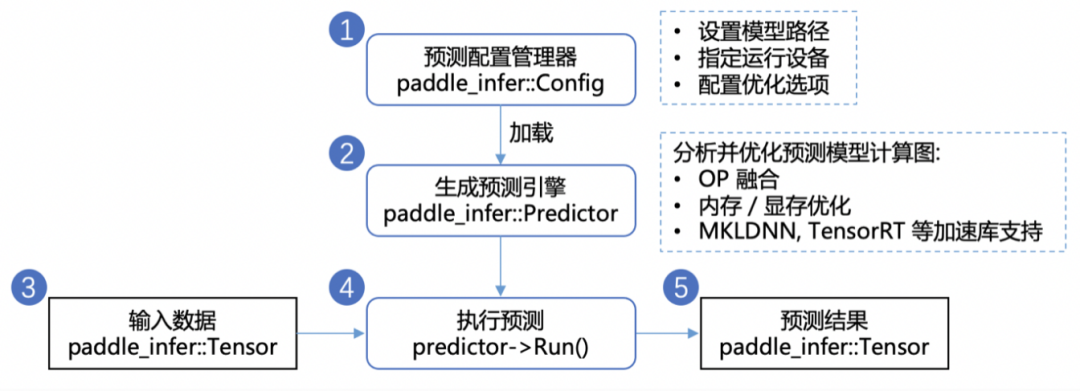``import paddle.inference as paddle_infer# 1. 创建配置对象，设置预测模型路径 config = paddle_infer.Config("infer_model/model.pdmodel", "infer_model/model.pdiparams")# 启用 GPU 进行预测 - 初始化 GPU 显存 100M, Deivce_ID 为 0# config.enable_use_gpu(100, 0)config.disable_gpu()# 2. 根据配置内容创建推理引擎predictor = paddle_infer.create_predictor(config)# 3. 设置输入数据# 获取输入句柄input_handles = [            predictor.get_input_handle(name)            for name in predictor.get_input_names()        ]# 获取输入数据data = dev_batchify_fn([dev_ds])# 设置输入数据for input_field, input_handle in zip(data, input_handles):    input_handle.copy_from_cpu(input_field)# 4. 执行预测predictor.run()# 5. 获取预测结果# 获取输出句柄output_handles = [            predictor.get_output_handle(name)            for name in predictor.get_output_names()        ]# 从输出句柄获取预测结果``

https://aistudio.baidu.com/aistudio/projectdetail/2114383END